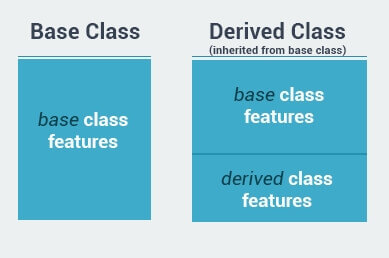## c++ 中 string 字符串的含义

``````int main()
{
char *s;
s = "hello";

cout << *s << endl;
cout << s << endl;

return 0;
}

//OUTPUT:
//h
//hello``````

``````int main()
{
int arr[] = {1, 3};
int *p = arr;

cout << *p << endl;
cout << *(p+1) << endl;

return 0;
}

//OUTPUT:
//1
//3``````

## c++ 中数组类型数据作为 function 参数时的注意事项

``````void testFunc(int arr[]) {
int count = sizeof(arr)/sizeof(arr);
}

int main()
{
int arr[] = {1, 3, 6, 8};
cout << testFunc(arr) << endl;

return 0;
}``````

sizeof function 可以返回数据占用内存空间的大小。所以用整个 arr 大小除以第一个元素大小可以得到数组元素个数。

- 阅读剩余部分 -

## C++ 入门教程之八 -- Templates - Exceptions - Files

### function template

``````int sum(int a, int b) {
return a+b;
}

int main () {
int x=7, y=15;
cout << sum(x, y) << endl;
}``````

- 阅读剩余部分 -

## C++ 入门教程之七 -- 继承和多态

### inheritance 继承

inheritance 继承是面向对象编程最重要的概念之一。继承允许我们基于已有的 class 创建新的 class。这能够极大的方便我们创建应用程序。- 阅读剩余部分 -

- 阅读剩余部分 -